Students can download Maths Chapter 5 Coordinate Geometry Ex 5.5 Questions and Answers, Notes, Samacheer Kalvi 10th Maths Guide Pdf helps you to revise the complete Tamilnadu State Board New Syllabus, helps students complete homework assignments and to score high marks in board exams.

## Tamilnadu Samacheer Kalvi 10th Maths Solutions Chapter 5 Coordinate Geometry Ex 5.5

Multiple Choice Questions

Question 1.
The area of triangle formed by the points (-5, 0), (0, – 5) and (5, 0) is …………..
(1) 0 sq.units
(2) 25 sq.units
(3) 5 sq.units
(4) none of these
(2) 25 sq.units Hint.
Hint:
Area of the ∆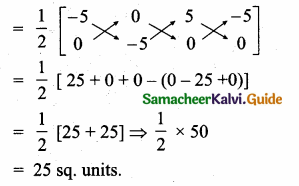To round a number to the nearest tenth, we need to look to the right of the tenths place, which is the hundredths place.

Question 2.
A man walks near a wall, such that the distance between him and the wall is 10 units. Consider the wall to be the Y axis. The path travelled by the man is …………
(1) x = 10
(2) y = 10
(3) x = 0
(4) y = 0
(1) x = 10
Hint: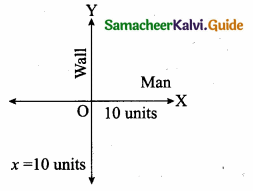Question 3.
The straight line given by the equation x = 11 is …………….
(1) parallel to X axis
(2) parallel to Y axis
(3) passing through the origin
(4) passing through the point (0,11)
(2) parallel to Y axisQuestion 4.
If (5,7), (3,p) and (6,6) are collinear, then the value of p is ……………
(1) 3
(2) 6
(3) 9
(4) 12
(3) 9
Hint:
Since the three points are collinear. Area of a triangle is 0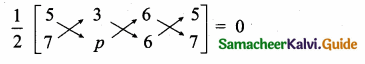5p + 18 + 42 – (21 + 6p + 30) = 0
5p + 60 – (51 + 6p) = 0
5p + 60 – 51 – 6p = 0
-p + 9 = 0
-p = -9
p = 9

Question 5.
The point of intersection of 3x – y = 4 and x + 7 = 8 is ……………
(1) (5,3)
(2) (2,4)
(3) (3,5)
(4) (4, 4)
(3) (3, 5)Substitute the value of x = 3 in (2)
3 + 7 = 8
y = 8 – 3 = 5
The point of intersection is (3, 5)Question 6.
The slope of the line joining (12, 3), (4, a) is $$\frac { 1 }{ 8 }$$. The value of ‘a’ is …………….
(1) 1
(2) 4
(3) -5
(4) 2
(4) 2
Hint:
Slope of a line = $$\frac { 1 }{ 8 }$$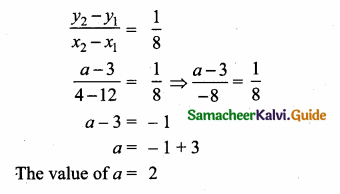Question 7.
The slope of the line which is perpendicular to a line joining the points (0, 0) and (- 8, 8) is ………..
(1) -1
(2) 1
(3) $$\frac { 1 }{ 3 }$$
(4) -8
(2) 1
Hint:
Slope of a line = $$\frac{y_{2}-y_{1}}{x_{2}-x_{1}}$$
= $$\frac { 8-0 }{ -8-0 }$$ = $$\frac { 8 }{ -8 }$$ = -1
Slope of the Perpendicular = 1

Question 8.
If slope of the line PQ is $$\frac{1}{\sqrt{3}}$$ then slope of the perpendicular bisector of PQ is …………..
(1) $$\sqrt { 3 }$$
(2) –$$\sqrt { 3 }$$
(3) $$\frac{1}{\sqrt{3}}$$
(4) 0
(2) –$$\sqrt { 3 }$$
Hint:
Slope of a line = $$\frac{1}{\sqrt{3}}$$
Slope of the ⊥r bisector = –$$\sqrt { 3 }$$Question 9.
If A is a point on the Y axis whose ordinate is 8 and B is a point on the X axis whose abscissae is 5 then the equation of the line AB is ……………
(1) 8x + 5y = 40
(2) 8x – 5y = 40
(3) x = 8
(4) y = 5
(1) 8x + 5y = 40
Hint:
Let the point A be (0, 8) and B (5, 0)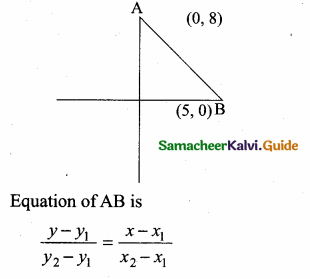Question 10.
The equation of a line passing through the origin and perpendicular to the line lx -3y + 4 = 0 is
(1) 7x – 3y + 4 = 0
(2) 3x – 7y + 4 = 0
(3) 3x + 7y = 0
(4) 7x – 3y = 0
(3) 3x + 7y = 0
Hint:
Slope of the line of 7x – 3y + 4 = 0
= $$\frac { -7 }{ -3 }$$ = $$\frac { 7 }{ 3 }$$
Slope of its ⊥r = $$\frac { -3 }{ 7 }$$
The line passes through (0,0)
Equation of a line is
y – y1 = m(x – x1)
y – 0 = $$\frac { -3 }{ 7 }$$ (x – 0)
y = $$\frac { -3 }{ 7 }$$ x ⇒ 7y = -3x
3x + 7y = 0Question 11.
Consider four straight lines
(i) l1 : 3y = 4x + 5
(ii) l2 : 4y = 3x – 1
(iii) l3 : 4y + 3x = 7
(iv) l4 : 4x + 3y = 2
Which of the following statement is true?
(1) l1 and l2 are perpendicular
(2) l2 and l4 are parallel
(3) l2 and l4 are perpendicular
(4) l2 and l3 are parallel
(3) l2 and l4 are perpendicular
Hint:
Slope of l1 = $$\frac { 4 }{ 3 }$$; Slope of l2 = $$\frac { 3 }{ 4 }$$
Slope of l3 = – $$\frac { 3 }{ 4 }$$; Slope of l4 = –$$\frac { 4 }{ 3 }$$
(1) l1 × l2 = $$\frac { 4 }{ 3 }$$ × $$\frac { 3 }{ 4 }$$ = 1 …….False
(2) l1 = $$\frac { 4 }{ 3 }$$; l4 = – $$\frac { 4 }{ 3 }$$ not parallel ………False
(3) l2 × l4 = $$\frac { 3 }{ 4 }$$ × – $$\frac { 4 }{ 3 }$$ = -1 …….True
(4) l2 = $$\frac { 3 }{ 4 }$$; l3 = – $$\frac { 3 }{ 4 }$$ not parallel ………FalseQuestion 12.
A straight line has equation 87 = 4x + 21. Which of the following is true …………………….
(1) The slope is 0.5 and the y intercept is 2.6
(2) The slope is 5 and the y intercept is 1.6
(3) The slope is 0.5 and they intercept is 1.6
(4) The slope is 5 and the y intercept is 2.6
(1) The slope is 0.5 and they intercept is 2.6
Hint:
8y = 4x + 21
y = $$\frac { 4 }{ 8 }$$ x + $$\frac { 21 }{ 8 }$$
= $$\frac { 1 }{ 2 }$$ x + $$\frac { 21 }{ 8 }$$
$$\frac { 1 }{ 2 }$$ = 0.5
$$\frac { 21 }{ 8 }$$ = 2.625
Slope = $$\frac { 1 }{ 2 }$$ = 0.5
y intercept = $$\frac { 21 }{ 8 }$$ = 2.6Question 13.
When proving that a quadrilateral is a trapezium, it is necessary to show
(1) Two sides are parallel.
(2) Two parallel and two non-parallel sides.
(3) Opposite sides are parallel.
(4) All sides are of equal length.
Solution:
(2) Two parallel and two non-parallel sides.

Question 14.
When proving that a quadrilateral is a parallelogram by using slopes you must find …………………
(1) The slopes of two sides
(2) The slopes of two pair of opposite sides
(3) The lengths of all sides
(4) Both the lengths and slopes of two sides